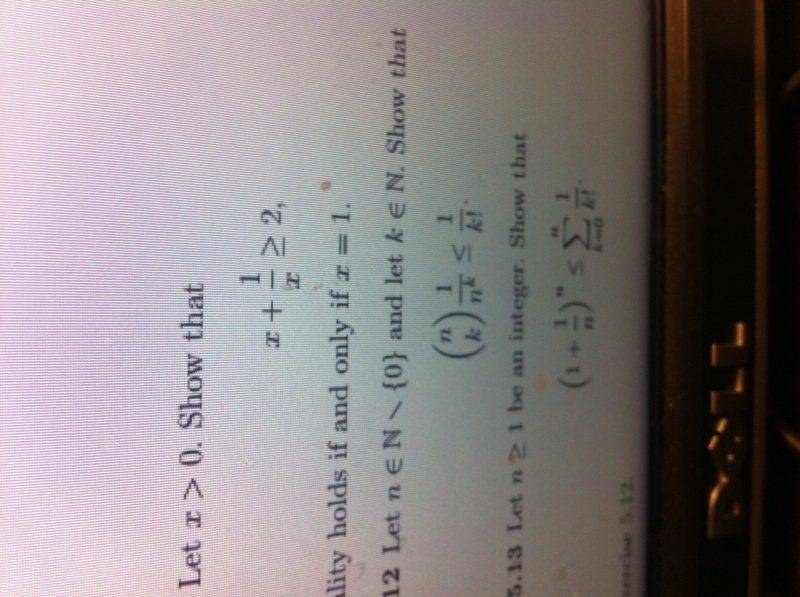# Inequality proof

## Homework Statementits the second one.
let n∈ℕ \ 0 and k∈ℕ show that
(n choose k) 1/n^k <= 1/k!

## Homework Equations

axioms of ordered fields?

## The Attempt at a Solution

[/B]i have been working on this all afternoon. I know 0<k<n since its a requirement for (n choose k). Ive tried induction, base case works but cannot figure out how to do inductive step. I dont really think induction is the correct method. Ive tried cases, n=k is easy to prove but n>k i cannot figure out. Any help getting me started in the right direction would be appreciated.

## Answers and Replies

As n > 0 you can instead try proving the equivalent statement:

$$\binom n k \le \frac {n^k}{k!}$$

RUber
Homework Helper
##n! < n^n ##
So what inequality might hold for the largest k terms of n! ?

Thanks for the replies.

I tried playing around with these for an hour this morning. When trying to prove that equivalent statement, Its obvious that its true but I still don't know how to "prove" it. Leaves me in the same situation as before just allows me to cancel a factor of 1/k! from either side of the inequality.

RUber, that inequality holds for all n>=2 i believe. Since k has to be less than n though the only way I could write k in terms of n is n-1 and at this point i am struggling again on what to do next.

Right now I'm thinking cases is the best way to try and prove this since its easy to prove the inequality for n=k. I just need to prove that it holds for n>k and then I would be done.

RUber
Homework Helper
I don't think you need induction, this is a straightforward relation.
##\left( \begin{array}{c} n\\k \end{array} \right) \frac{1}{n^k} \leq \frac{1}{k!} ##
Is saying:
##\frac{n!}{(n-k)!(k)!}\frac{1}{n^k} \leq \frac{1}{k!} ##
Which is equivalent to (for k >0)
##\frac{\left( \prod_{i = n-k+1}^n i \right) }{(k)!}\frac{1}{n^k} \leq \frac{1}{k!} ##
So as you said by cancelling out the ##\frac{1}{k!} ## the relation you need to prove is :
##\frac{\left( \prod_{i = n-k+1}^n i \right) }{n^k} \leq 1##
And for k= 0 :
##\frac{n!}{(n-k)!(k)!}\frac{1}{n^k} =1 \leq \frac{1}{k!} =1 ##

ok i'm able to understand most of this. Im a little confused how you changed n!/(n-k)! into that product but i can see that it makes sense. Isn't k=0 just one case though? Wouldn't i still need to prove somehow that the inequality holds for k>0 but still k<n?

RUber
Homework Helper
## \frac{5!}{(5-2)!}= \frac{5*4*3*2*1}{3*2*1}=\prod_{i=5-2+1}^5 i =\prod_{i=4}^5 i=4*5.##
The first relation I wrote above (with the product notation) is for any k not equal to zero, then for k=0, the relation is just 1=1.
I find it odd that it seems your definition of natural numbers includes zero. That is not what I am used to. In any case, it just adds one case to the proof.

Ok thanks im pretty sure I got it now.

Ray Vickson
Science Advisor
Homework Helper
Dearly Missed

## Homework Statement

View attachment 74442

its the second one.
let n∈ℕ \ 0 and k∈ℕ show that
(n choose k) 1/n^k <= 1/k!

## Homework Equations

axioms of ordered fields?

## The Attempt at a Solution

[/B]
i have been working on this all afternoon. I know 0<k<n since its a requirement for (n choose k). Ive tried induction, base case works but cannot figure out how to do inductive step. I dont really think induction is the correct method. Ive tried cases, n=k is easy to prove but n>k i cannot figure out. Any help getting me started in the right direction would be appreciated.

If you insist on posting images (which is discouraged in PF) you should at least attempt to post them correctly--not sideways like you did. If you want help, don't make it difficult for us to see what you are doing.